﻿ Set Of Circle Percentage Diagrams For Infographics, Stock Vector - Illustration of background, diagram: 85701841

Set of circle percentage diagrams for infographics, .

Set of circle percentage diagrams for infographics, 0 5 10 15 20 25 30 35 40 45 50 55 60 65 70 75 80 85 90 95 100 percent

Designers Also Selected These Stock IllustrationsPay with Cell Phone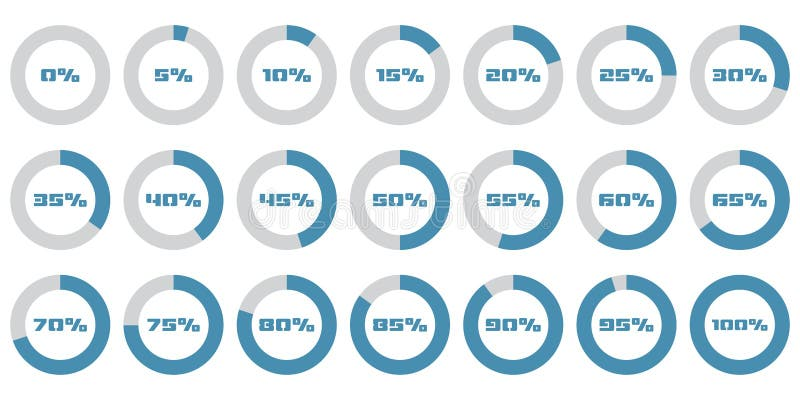Set of circle percentage diagrams for infographics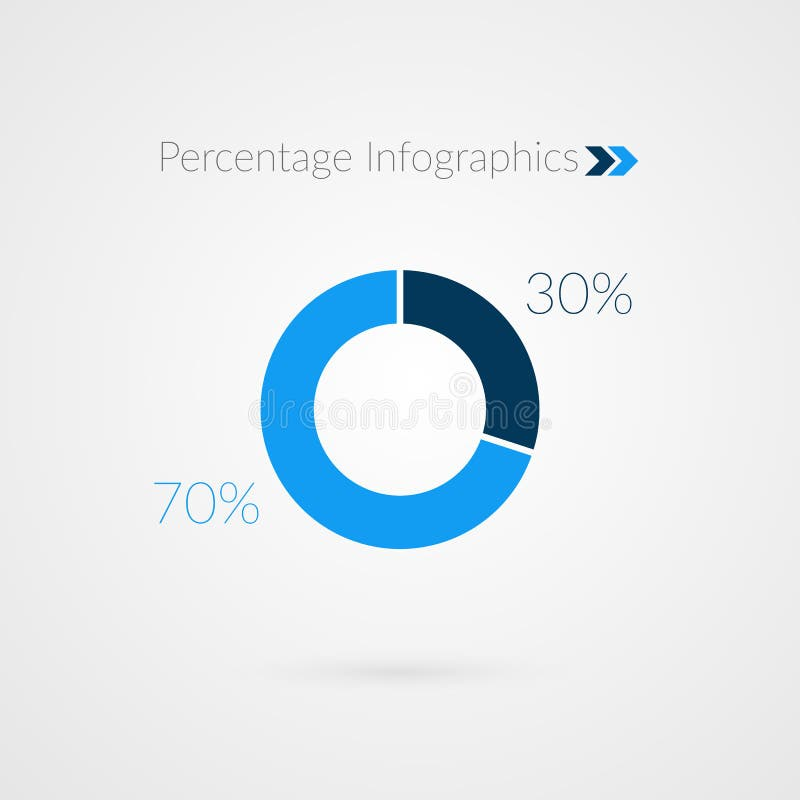30 70 percent blue pie chart symbol. Percentage vector infographics. Circle diagram isolated
More similar stock illustrations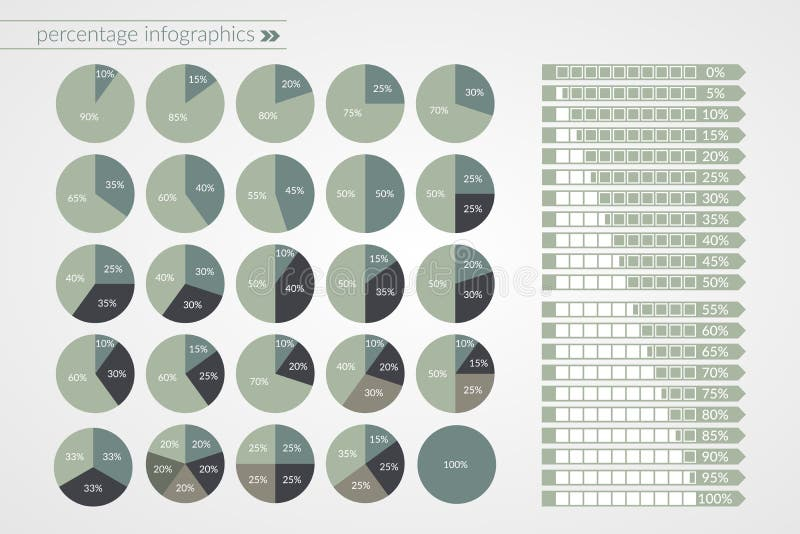0 to 100 percent pie and arrow charts. Vector percentage infographics. Circle and line diagrams isolated. Set of business icons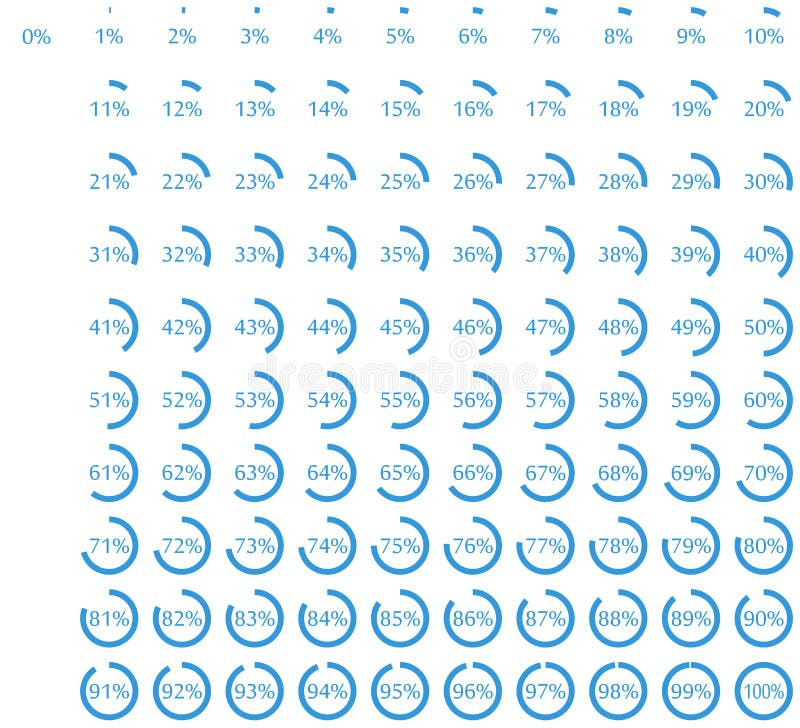Set of circle percentage diagrams for infographics. Vector illustration.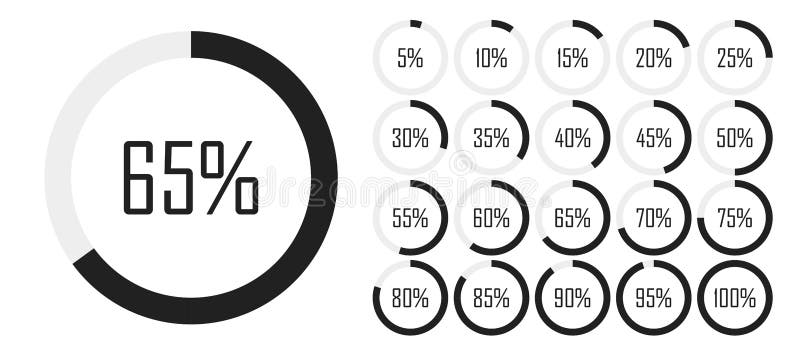Set of circle percentage diagrams from 0 to 100 for infographics, lignt, 5 10 15 20 25 30 35 40 45 50 55 60 65 70 75 80 85 90 95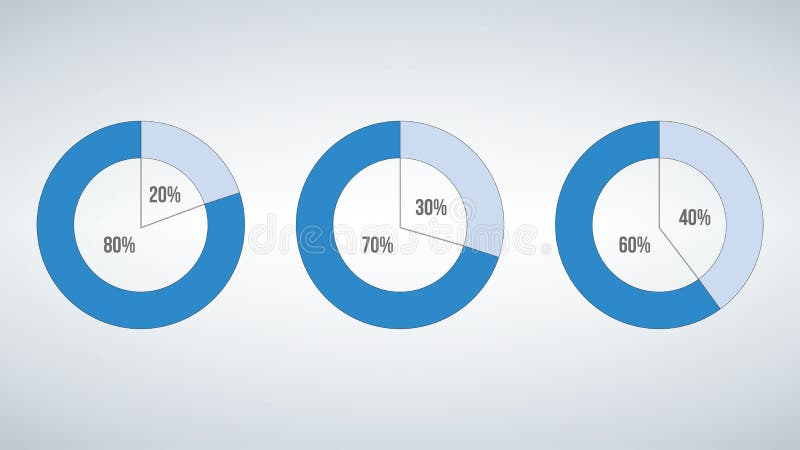Blue Set of circle percentage diagrams for infographics. Vector illustration isolated on modern background.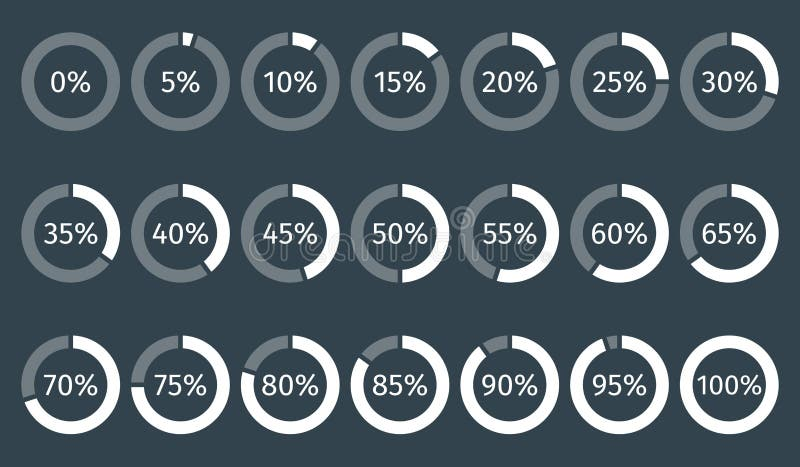Set of circle percentage diagrams for infographics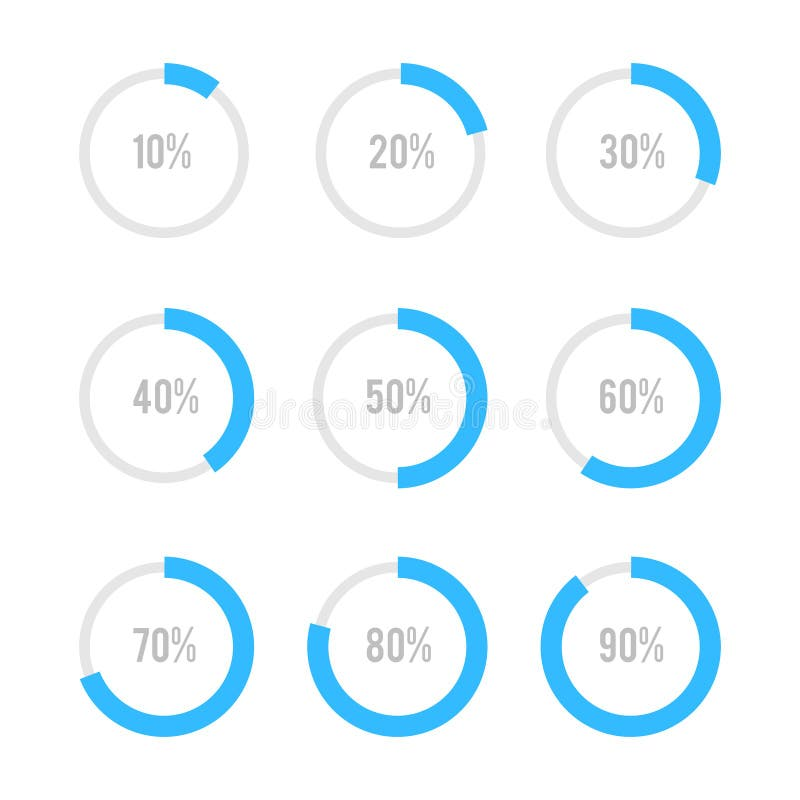Set of circle diagrams for infographics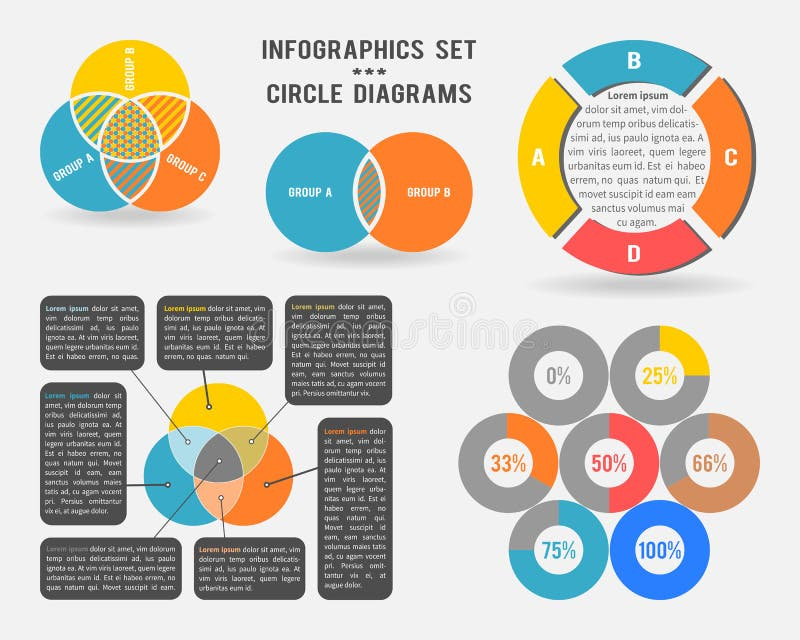Vector infographics set of circle diagrams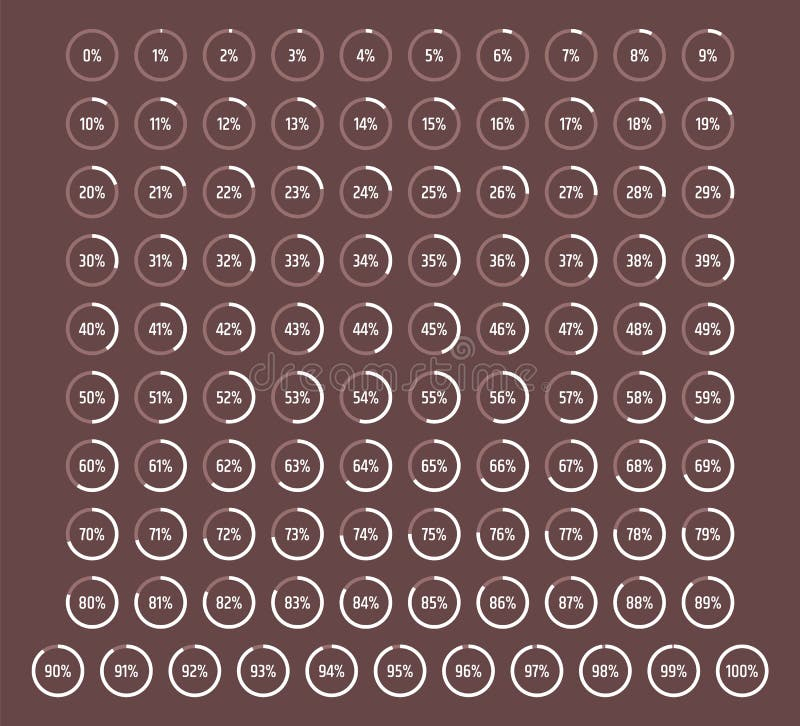Set of circle percentage diagrams from 0 to 100, vector percentage infographics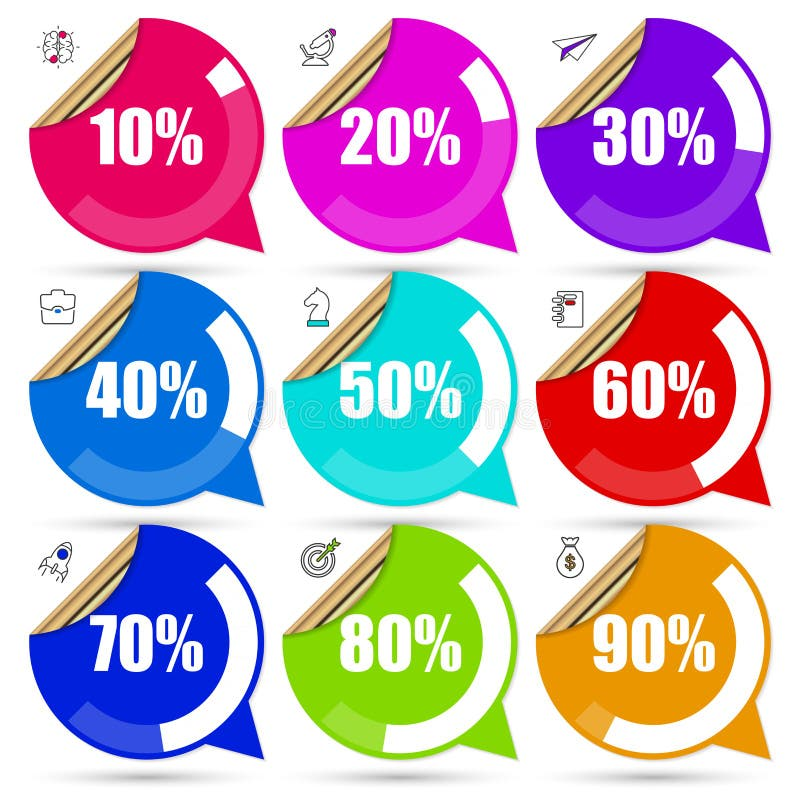Set of percentage diagrams for Infographics design. Vector
More stock photos from Stas11's portfolio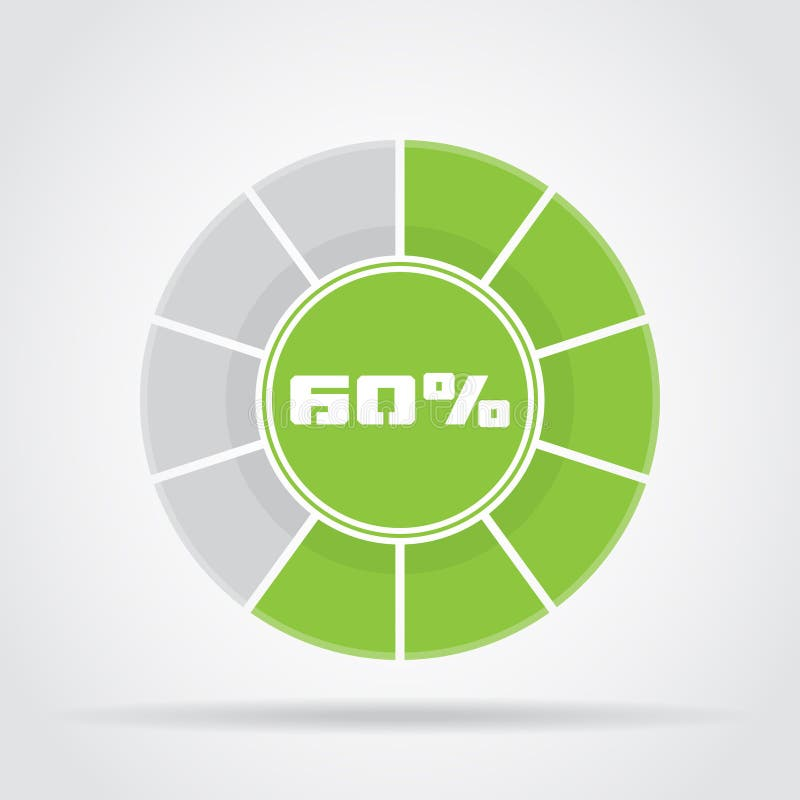Green pie chart percentage diagram with shadow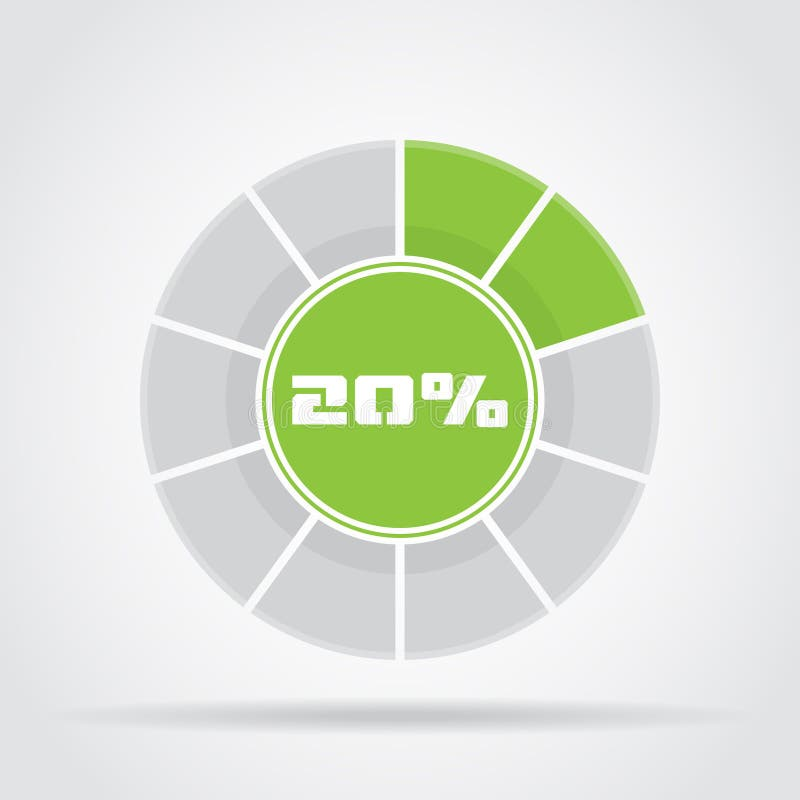Green pie chart percentage diagram with shadow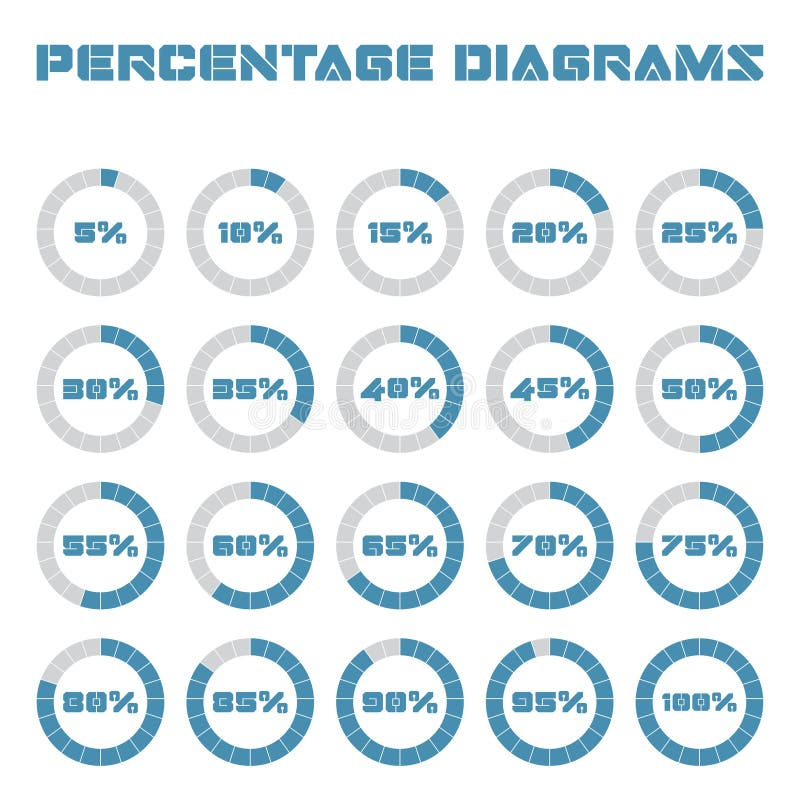Set of circle percentage diagrams for infographics, 5 10 15 20 25 30 35 40 45 50 55 60 65 70 75 80 85 90 95 100 percent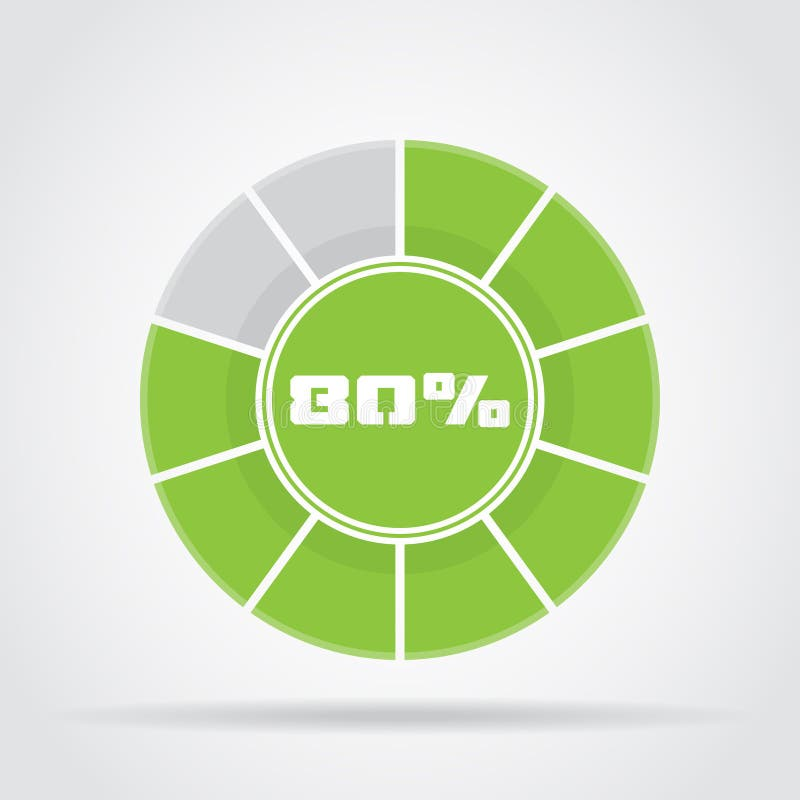Green pie chart percentage diagram with shadow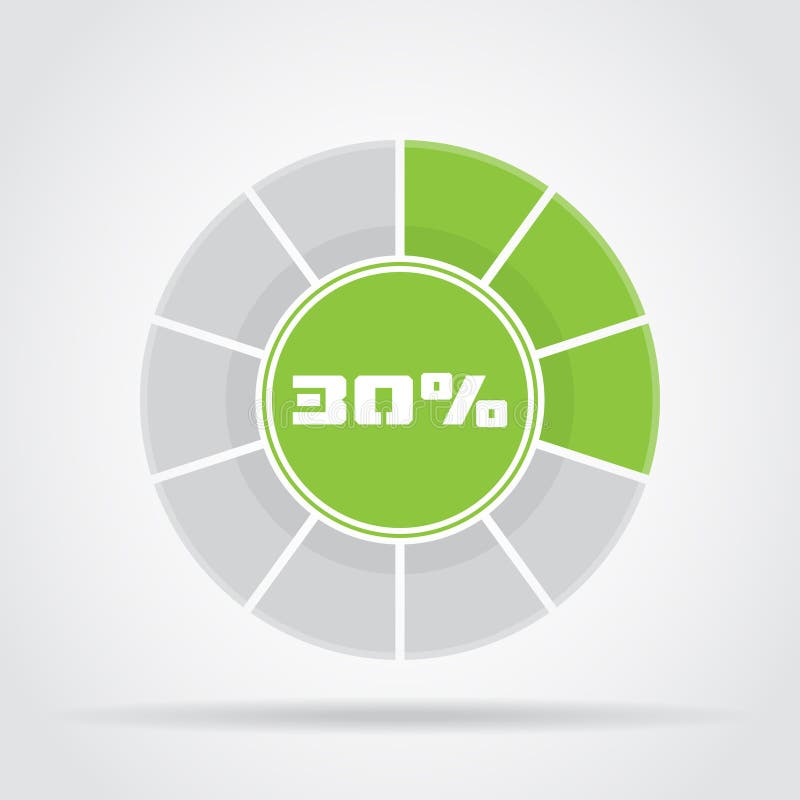Green pie chart percentage diagram with shadow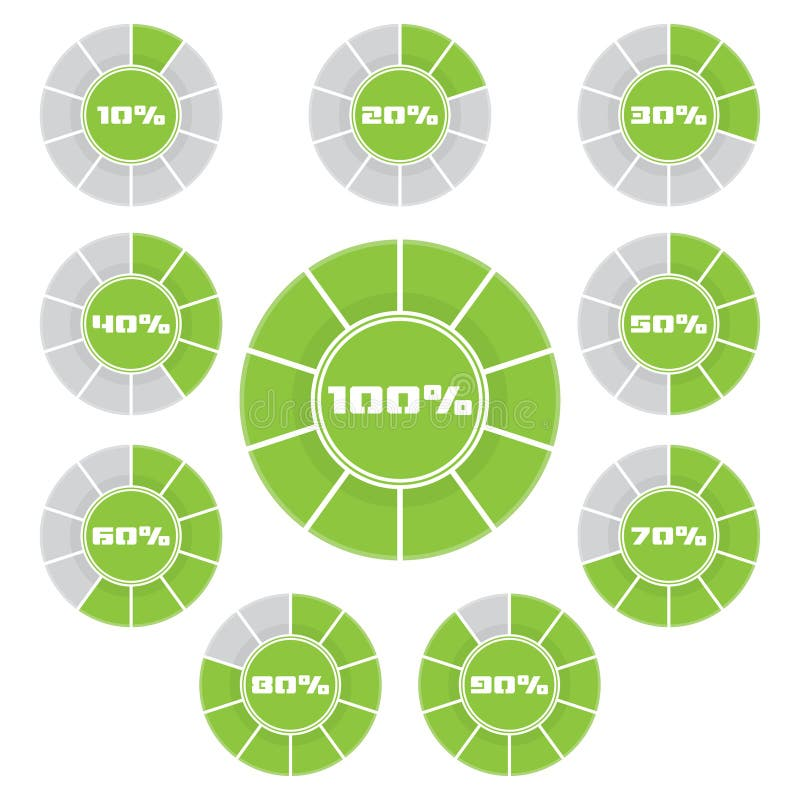Set of green pie chart percentage diagram with shadow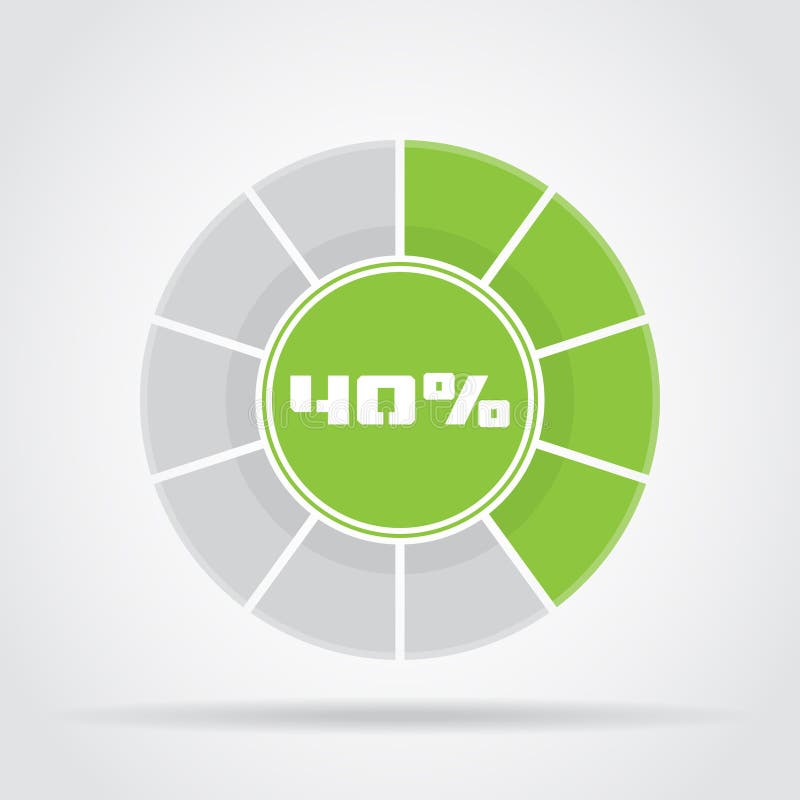Green pie chart percentage diagram with shadow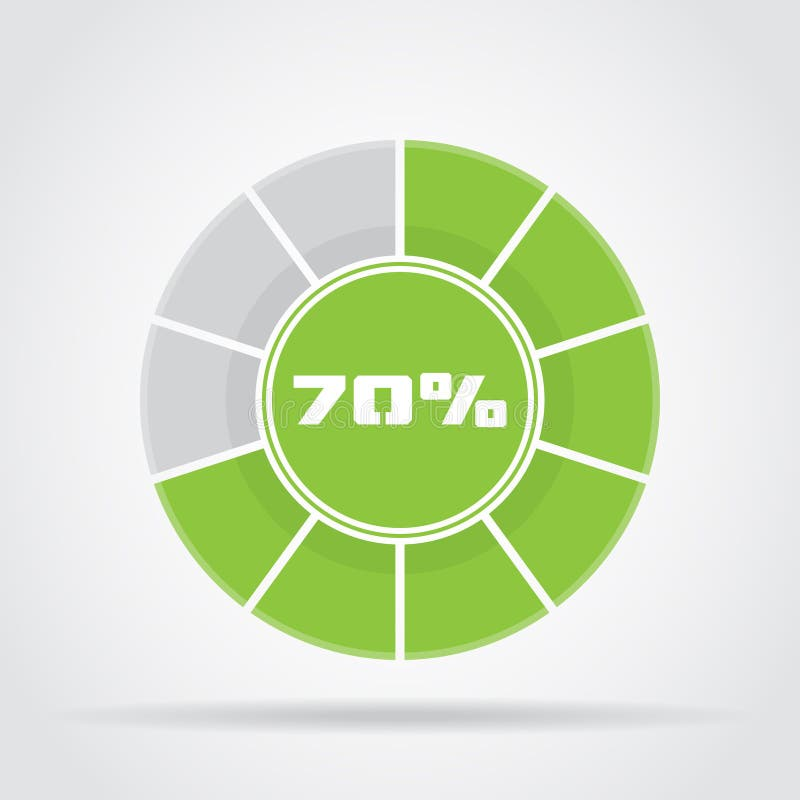Green pie chart percentage diagram with shadow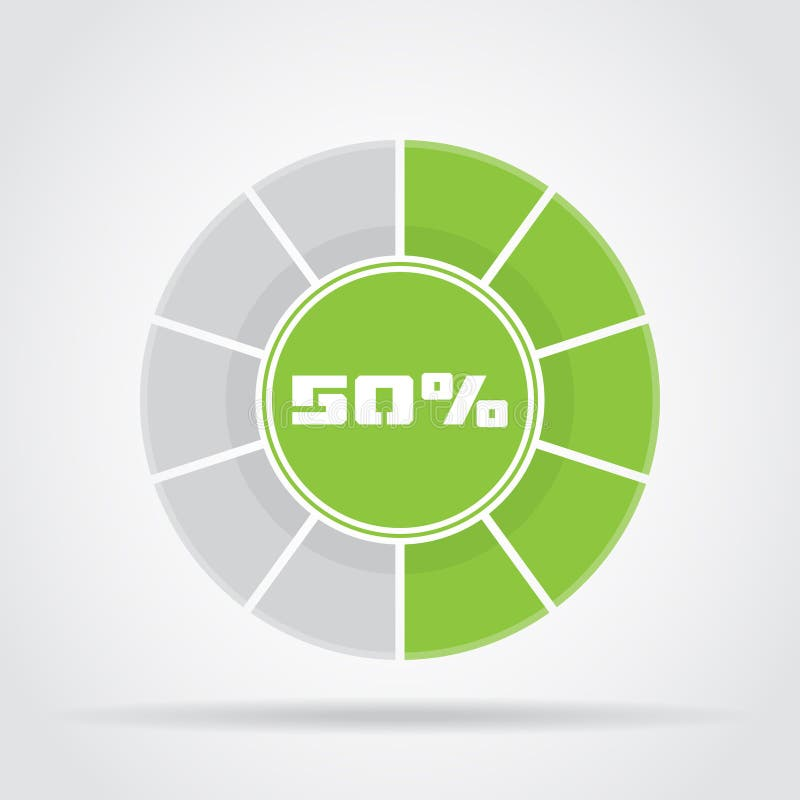Green pie chart percentage diagram with shadow
Related categories